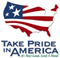Techniques and Methods

# UCODE_2005 and Six Other Computer Codes for Universal Sensitivity Analysis, Calibration, and Uncertainty Evaluation

This report is available as a pdf.

## ABSTRACT

This report documents the computer codes UCODE_2005 and six post-processors. Together the codes can be used with existing process models to perform sensitivity analysis, data needs assessment, calibration, prediction, and uncertainty analysis.  Any process model or set of models can be used; the only requirements are that models have numerical (ASCII or text only) input and output files, that the numbers in these files have sufficient significant digits, that all required models can be run from a single batch file or script, and that simulated values are continuous functions of the parameter values.  Process models can include pre-processors and post-processors as well as one or more models related to the processes of interest (physical, chemical, and so on), making UCODE_2005 extremely powerful.  An estimated parameter can be a quantity that appears in the input files of the process model(s), or a quantity used in an equation that produces a value that appears in the input files.  In the latter situation, the equation is user-defined.

UCODE_2005 can compare observations and simulated equivalents. The simulated equivalents can be any simulated value written in the process-model output files or can be calculated from simulated values with user-defined equations. The quantities can be model results, or dependent variables. For example, for ground-water models they can be heads, flows, concentrations, and so on. Prior, or direct, information on estimated parameters also can be considered. Statistics are calculated to quantify the comparison of observations and simulated equivalents, including a weighted least-squares objective function. In addition, data-exchange files are produced that facilitate graphical analysis.

UCODE_2005 can be used fruitfully in model calibration through its sensitivity analysis capabilities and its ability to estimate parameter values that result in the best possible fit to the observations. Parameters are estimated using nonlinear regression: a weighted least-squares objective function is minimized with respect to the parameter values using a modified Gauss-Newton method or a double-dogleg technique.  Sensitivities needed for the method can be read from files produced by process models that can calculate sensitivities, such as MODFLOW-2000, or can be calculated by UCODE_2005 using a more general, but less accurate, forward- or central-difference perturbation technique. Problems resulting from inaccurate sensitivities and solutions related to the perturbation techniques are discussed in the report. Statistics are calculated and printed for use in (1) diagnosing inadequate data and identifying parameters that probably cannot be estimated; (2) evaluating estimated parameter values; and (3) evaluating how well the model represents the simulated processes.

Results from UCODE_2005 and codes RESIDUAL_ANALYSIS and RESIDUAL_ANALYSIS_ADV can be used to evaluate how accurately the model represents the processes it simulates. Results from LINEAR_UNCERTAINTY can be used to quantify the uncertainty of model simulated values if the model is sufficiently linear. Results from MODEL_LINEARITY and MODEL_LINEARITY_ADV can be used to evaluate model linearity and, thereby, the accuracy of the LINEAR_UNCERTAINTY results.

UCODE_2005 can also be used to calculate nonlinear confidence and predictions intervals, which quantify the uncertainty of model simulated values when the model is not linear. CORFAC_PLUS can be used to produce factors that allow intervals to account for model intrinsic nonlinearity and small-scale variations in system characteristics that are not explicitly accounted for in the model or the observation weighting.

The six post-processing programs are independent of UCODE_2005 and can use the results of other programs that produce the required data-exchange files.

UCODE_2005 and the other six codes are intended for use on any computer operating system. The programs consist of algorithms programmed in Fortran 90/95, which efficiently performs numerical calculations.  The model runs required to obtain perturbation sensitivities can be performed using multiple processors. The programs are constructed in a modular fashion using JUPITER API conventions and modules. For example, the data-exchange files and input blocks are JUPITER API conventions and many of those used by UCODE_2005 are read or written by JUPITER API modules. UCODE-2005 includes capabilities likely to be required by many applications (programs) constructed using the JUPITER API, and can be used as a starting point for such programs.

## CONTENTS

Abstract

Chapter 1:  Introduction

Chapter 2:  Overview and Program Control

Chapter 3:  User Considerations

Chapter 4:  Running UCODE_2005, Residual_Analysis, Model_Linearity, and Linear-Uncertainty

Chapter 5:  Overview of UCODE_2005 Input Instructions

Chapter 6:  Input to Control UCODE_2005 Operation

Chapter 7:  Input to Define Parameters

Chapter 8:  Input to Define Observations and Predictions

Chapter 9:  Input to Include Measurements of Parameter Values

Chapter 10:  Input to Define Weight Matrices

Chapter 11:  Input to Interact with the Process Model Input and Output Files

Chapter 12:  Input for Parallel Execution

Chapter 13:  Equation Protocols and Two Additional Input Files

Chapter 14:  UCODE_2005 Output Files

Chapter 15:  Evaluation of Residuals, Nonlinearity, and Uncertainty

Chapter 16:  Use of Output from UCODE_2005, Residual_Analysis, Model_Linearity, and Linear_Uncertainty

Chapter 17:  Nonlinear Confidence Intervals and Advanced Evaluation of Residuals and Nonlinearity

Chapter 18:  References

Appendix A.  Connection with the JUPITER.API

Appendix B.  Files Produced by Using the Filename Prefix Specified on Command Lines

Appendix C.  Example Simulation

Appendix D.  Program Distribution and Installation

Appendix E.  Comparison with UCODE as Documented by Poeter and Hill (1998)

Appendix F.  Abbreviated Input Instructions for UCODE_2005

Appendix G.  Abbreviated Input Instructions for Other Codes

This report is available online in Portable Document Format (PDF). If you do not have the Adobe Acrobat PDF Reader, it is available for free download from Adobe Systems Incorporated.

 AccessibilityFOIAPrivacyPolicies and Notices U.S. Department of the Interior, U.S. Geological Survey Persistent URL: Page Contact Information: Contact USGS Last modified: Friday, December 02 2016, 03:43:53 PM